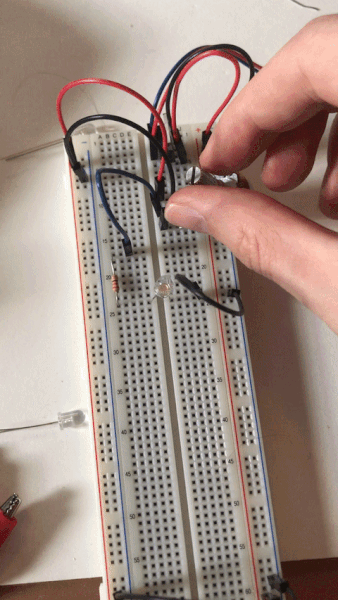# Lab: Electronics

September 13, 2020

Physical Computing

Lab Guide

In this lab I will learn how to use the multimeter to measure continuity, resistance, voltage, and amperage.

## Measuring Continuity

Continuity means that two points are electrically connected. I looked for continuity in a wires, a switch, the breadboard, and my hand.

This wire is continuous

Measuring a switch:

This switch is not continuous in it’s unpressed state

This switch is continuous when pressed

These two points are not continuous

These two points are continuous

## Measuring Resistance

Resistance is the measurement of how much a certain path resists electrical current. This 220Ω resistor showed an actual resistance of 215Ω.

Measuring a 220Ω resistor

The resistance across my hand fluctuated around 5MΩ.

Measuring resistance across my hand

## Measuring Voltage

Voltage is a measurement of electrical potential between to points. To measure voltage I created this simple circuit: A 220Ω resistor in series with an LED. The voltage measured from power to ground is 4.80V.

Measuring the voltage between power and ground

The voltage drop across the LED is 2.89V. I got a negative value because the black lead touches the point of higher voltage.

Measuring the voltage across the LED

I then modified the circuit by adding a pushbutton in series before the resistor and LED.

The circuit with a pushbutton added in series before the resistor

The LED lights up when the button is pressed

I measured the voltage drop across the pushbutton to be 2.66V when not pressed and 0V when pressed. This confused me a bit because I thought that the act of measuring the button’s voltage would bypass the button and complete the circuit. I noticed that the LED illuminated slightly which means that the circuit was indeed complete, but only a small voltage was reaching the LED.

Measuring the voltage drop across the unpressed button: 2.66V

Measuring the voltage drop across the pressed button: 0V

When the button was pressed, the voltage drops across the resistor and LED were each 0V because the circuit was incomplete. When the button was pressed, the drop across the resistor was 2.15V and the voltage across the LED was 2.85. These add up to the 5V total voltage drop across the circuit.

For the next circuit I added second LED in series with the first:

The circuit with a second LED

Pressing the button causes both LEDs to illuminate

Pressing the button causes both LEDs to illuminate. The first consumed 2.46V and the second consumed 2.52V. The voltage across the resistor was just 0.01V because the LEDs were drawing the rest of the voltage. Added up, the voltage drop is 4.99V. The remaining 0.01V could be attributed to measurement fidelity or a slight loss of energy to light or heat.

Adding a third LED in series with the other two causes none of the LEDs to light up when the button is pressed. This is because they each require about 2V, but the available power is only 5V. Because they are in series, each LED only receives about 1.67V.

Pressing the button does not turn on the LEDs

The next circuit orients the three LEDs in parallel:

Three LEDs in parallel illuminate when the button is pressed

The voltage drop across is the same across each LED: 2.72V, and 2.21V across the resistor. This works because the voltage is not divided across the three LEDs. sss

## Measuring Amperage

Amperage is the unit of electrical current. I had trouble measuring the amperage across the LED in my circuit. I connected the multimeter’s red lead to the resistor’s output and the black lead the the LED’s anode, and the LED’s cathode to ground. When I pressed the button, all three LEDs lit up but I did not see any amperage value on the multimeter.

Using the multimeter in series with the LED

The multimeter displayed 0A

## Generating a Variable Voltage with a Potentiometer

For the final step I built a circuit that uses a potentiometer to control the voltage across the LED. The voltage drop goes from 0 to 5 as the potentiometer is rotated.